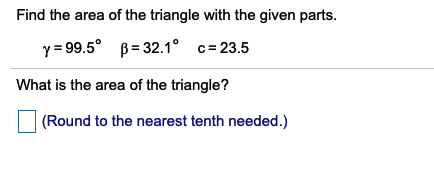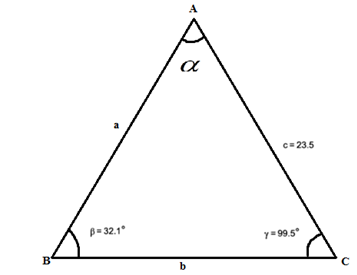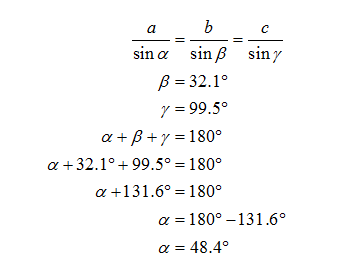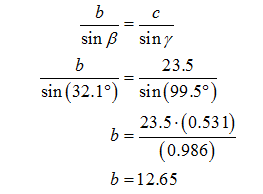# Find the area of the triangle with the given parts.y = 99.5° B= 32.1° c=23.5What is the area of the triangle?(Round to the nearest tenth needed.)

Question
1 viewshelp_outlineImage TranscriptioncloseFind the area of the triangle with the given parts. y = 99.5° B= 32.1° c=23.5 What is the area of the triangle? (Round to the nearest tenth needed.) fullscreen
check_circle

Step 1

Consider the triangle ABC,Step 2

Use the sine rule and find unknown angles and sides.Step 3

Find the side "b"....

### Want to see the full answer?

See Solution

#### Want to see this answer and more?

Solutions are written by subject experts who are available 24/7. Questions are typically answered within 1 hour.*

See Solution
*Response times may vary by subject and question.
Tagged in